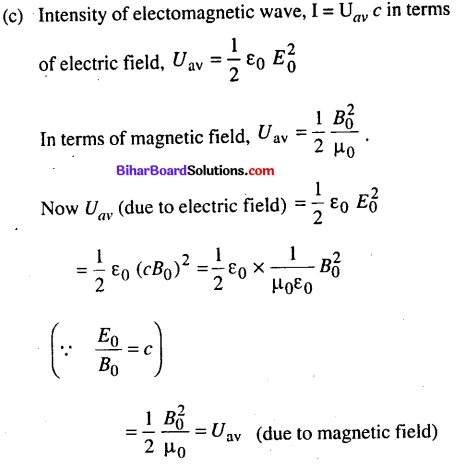# Bihar Board 12th Physics Objective Answers Chapter 8 Electromagnetic Waves

Bihar Board 12th Physics Objective Questions and Answers

## Bihar Board 12th Physics Objective Answers Chapter 8 Electromagnetic Waves

Question 1.
Maxwell in his famous equations of electromagnetism introduced the concept of
(a) ac current
(b) displacement current
(c) impedance
(d) reactance
(b) displacement current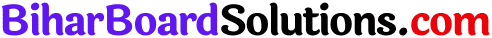Question 2.
Displacement current goes through the gap between the plates of a capacitor when the charge on the capacitor
(a) is changing with time
(b) decrease
(c) does not change
(d) decreases to zero
(a) is changing with time

Question 3.
The conduction current is same as displacement current when source is
(a) ac only
(b) dc only
(c) either ac or dc
(d) neither dc nor ac
(c) either ac or dc

Question 4.
Which among the following does not represent Maxwell’s equation ?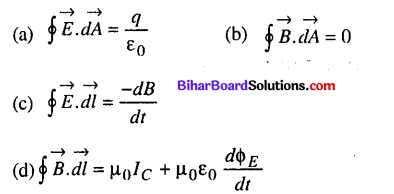(c) $$\oint \vec{E} \cdot \overrightarrow{d l}=\frac{-d B}{d t}$$
Solution:
Maxwell’s equation are as follows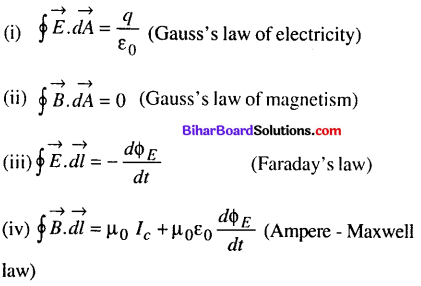Question 5.
An electromagnetic wave can be produced, when charge is
(a) moving with a constant velocity
(b) moving in a circular orbit
(c) falling in an electric field
(d) both (b) and (c)
(d) both (b) and (c)

Question 6.
If µ0 be the permeability and ε0 be the permittivity of a medium, then its refractive index is given by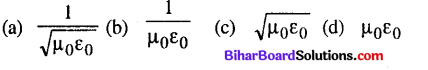(c) $$\sqrt{\mu_{0} \varepsilon_{0}}$$

Question 7.
A plane electromagnetic wave travels in vacuum along z-direction. If the frequency of the wave is 40 MHz then its wavelength is
(a) 5 m
(b) 7.5 m
(c) 8.5 m
(d) 10 m
(b) 7.5 m

Question 8.
A radio can tune to any station in 7.5 MHz to 12 MHz band. The corresponding wavelength band is
(a) 40 m to 25 m
(b) 30 m to 25 m
(c) 25 m to 10 m
(d) 10 m to 5 m
(a) 40 m to 25 m
Solution: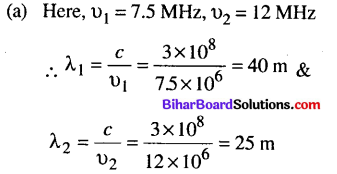Question 9.
Which of the following has/have zero average value in a plane electromagnetic wave ?
(a) Both magnetic & electric fields
(b) Electric field only
(c) Magnetic field only
(d) None of these
(a) Both magnetic & electric fields

Question 10.
A plane elecgtromagnetic wave propagating along x direction can be have the following pairs of $$\vec{E}$$ and $$\vec{E}$$
(a) Ey Bz
(b) Ez By
(c) Ex, By
(d) both (a) & (b)
(d) both (a) & (b)

Question 11.
The refractive index and permeability of a medium are 1.5 and 5 x 10-7 H m-1 respectively. The relative permittivity of the medium is nearly
(a) 25
(b) 15
(c) 10
(d) 6
(d) 6
Solution: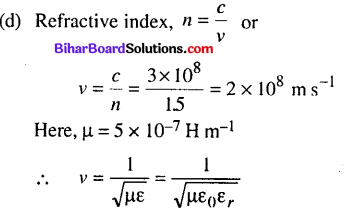Question 12.
If E and B denote electric and magnetic fields respectively, which of the following is dimensionless ?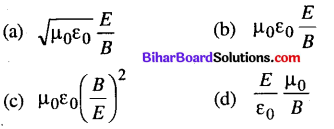(a) $$\sqrt{\mu_{0} \varepsilon_{0}} \frac{E}{B}$$

Question 13.
An electromagnetic wave propagating along north has its electric field vector upwards. Its magnetic field vector point towards
(a) north
(b) east
(c) west
(d) downwards
(b) east

Question 14.
The amplitude of the magnetic field of a harmonic
electromagnetic wave in vacuum is B0 = 510 nT. The amplitude of the electric field part of the wave is
(a) 120 NC-1
(b) 134 NC-1
(c) 510 NC-1
(d) 153NC-1
(d) 153NC-1Question 15.
The photon energy in units of eV for electromagtnetic waves of wavelength 2 cm is
(a) 2.5 x 10-19
(b) 5.2 x 1016
(c) 3.2 x 10-16
(d) 6.2 x 10-5
(d) 6.2 x 10-5
Solution: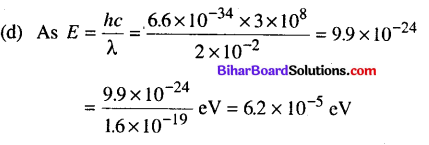Question 16.
The frequency of electromagnetic wave which is best suitable to observe a particle of radius 3 x 10″4 cm is of
the order of
(a) 1015 Hz
(b) 1014 Hz
(c) 1013 Hz
(d) 1012 Hz
(a) 1015 Hz
(a) Let λ be the radius of the particle then
λ = 3 x 10-4 x 10-2 m = 3 x 10-6 m
Frequency of electromagnetic wave,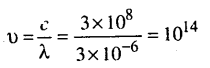Thus to observe the particle, the frequency of wave should be more than 1014 i.e. 1015 Hz or smaller value of wavelength.

Question 17.
A plane electromagnetic wave is incident on a material surface. The wave delivers momentum P and energy E. Then
(a) p ≠ 0, E ≠ 0
(b) p = 0,E = 0
(c) p = 0,E ≠ 0
(d) p ≠ 0,E = 0
(a) p ≠ 0, E ≠ 0Question 18.
Radiations of intensity 0.5 W m-2 are striking a metal plate. The pressure on the plate is
(a) 0.166 x 10-8 N m-2
(b) 0.332 x 10-8 N m-2
(c) 0.111 x 10-8 N m-2
(d) 0.083 x 10-8 N m-2
(a) 0.166 x 10-8 N m-2

Question 19.
Which of the following rays is not an electromagnetic wave ?
(a) X-rays
(b) γ-rays
(c) β-rays
(d) Heat rays
(c) β-rays

Question 20.
Radio waves diffract around buildings, although light waves do not. The reason is that radio waves
(a) travel with speed larger than c
(b) have much larger wavelength than light
(c) are not electromagnetic waves
(d) none of these
(b) have much larger wavelength than light

Question 21.
The ultra high frequency band of radiowaves in electromagnetic wave is used as in
(a) television waves
(b) cellular phone communication
(d) both (a) and (c)
(b) cellular phone communicationQuestion 22.
The part of the spectrum of the electromagnetic radiation used to cook food is
(a) ultraviolet rays
(b) cosmic rays
(c) Xrays
(d) microwaves
(d) microwaves

Question 23.
A microwave and an ultrasonic sound wave have the same wavelength. Their frequencies are in the ratio (approximately)
(a) 102
(b) 104
(c) 106
(d) 108
(c) 106

Question 24.
The waves used by artificial satellites for communication is
(a) microwaves
(b) infrared waves
(d) X-rays
(a) microwaves

Question 25.
Which waves are used in sonography ?
(a) Microwaves
(b) Infraredrays
(d) Ultrasonic waves
(d) Ultrasonic wavesQuestion 26.
Which of the following electromagnetic wave is used in high precision application like LASIK eye surgery ?
(a) Microwave
(b) Ultraviolet rays
(c) Gamma rays
(d) X-rays
(b) Ultraviolet rays

Question 27.
The crystal structure can be studied by using
(a) UVrays
(b) X-rays
(d) Microwaves
(b) X-rays

Question 28.
An electromagnetic radiation has an energy of 13.2 keV. Then the radiation belongs to the region of
(a) visible light
(b) ultraviolet
(c) infrared
(d) X-ray
(d) X-rayQuestion 29.
Which of the following electromagnetic waves has smallest wavelength ?
(a) X-rays
(b) Microwaves
(c) y-rays
(c) y-rays

Question 30.
Which of the following electromagnetic waves is used in medicine to destroy cancer cells ?
(a) IR-rays
(b) Visible rays
(c) Gamma rays
(d) Ultraviolet rays
(c) Gamma rays

Question 31.
The decreasing order of wavelength of infrared, microwave, ultraviolet and gamma rays is
(a) microwave, infrared, ultraviolet, gamma rays
(b) infrared, microwave, ultraviolet, gamma rays
(c) gamma rays, ultraviolet, infrared, microwaves
(d) microwaves, gamma rays, infrared, ultraviolet
(a) microwave, infrared, ultraviolet, gamma rays

Question 32.
X-rays and y-rays of same energies are distinguished by their
(a) frequency
(b) charges
(c) ionising power
(d) method of production
(d) method of production

Question 33.
If vg, vx and vm are speeds of gamma rays, X-rays and microwaves respectively in vacuum, then
(a) vg < vx < vm
(b) vg > vx > vm
(c) vg > vx < vm
(d) vg = vx = vm
(d) vg = vx = vmQuestion 34.
X-rays, gamma rays and microwaves travelling in vacuum have
(a) same wavelength but different velocities
(b) same frequency but different velocities
(c) same velocity but different wavelengths
(d) same velocity and same frequency
(c) same velocity but different wavelengths

Question 35.
If $$\vec{E}$$ and $$\vec{B}$$ represent electric and magnetic field vectors of the electromagnetic wave, the direction of propagation of electromagnetic wave is along
(a) $$\vec{E}$$
(b) $$\vec{B}$$
(c) $$\vec{B} \times \vec{E}$$
(d) $$\vec{E} \times \vec{B}$$
(d) $$\vec{E} \times \vec{B}$$Question 36.
The ratio of contributions made by the electric field and magnetic field components to the intensity of an electromagnetic wave is
(a) c : 1
(b) c2 : 1
(c) 1 : 1
(d) √c : 1